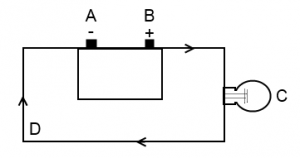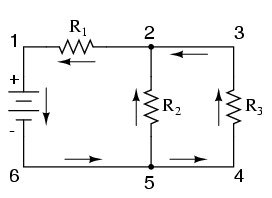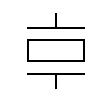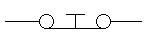# ASVAB Electronics Practice Test

Take our free ASVAB Electronics Information Practice Test. This test consists of 16 questions which must be completed within 8 minutes. This section of the ASVAB is designed to test your knowledge of electronics, electricity, electrical components, circuits, and electric formulas. Start your test prep right now with our Electronics Information ASVAB questions.

Congratulations - you have completed . You scored %%SCORE%% out of %%TOTAL%%. Your performance has been rated as %%RATING%%
 Question 1

### What does the letter I represent in this formula?

 A watts B voltage C current D impedance
Question 1 Explanation:
The correct answer is (C). The letter P represents power, which is measured in watts. The letter I represents current, which is measured in amperes. The letter V represents voltage which is measured in volts.
 Question 2

### What is the load in this basic circuit model?A A B B C C D D
Question 2 Explanation:
The correct answer is (C). In a basic circuit model, there is a voltage source (pictured here as a battery at points A and B), a load, and conductors. The conductors are drawn using the lines and arrows. The load in this model is the light bulb pictured at point C. Examples of loads are light bulbs, resistors and motors.
 Question 3

### Three amps equals how many milliamps?

 A 0.003 B 30 C 300 D 3,000
Question 3 Explanation:
The correct answer is (D). A milliamp is one thousandth of an amp. So every amp equals 1,000 milliamps:
3 × 1,000 = 3,000
 Question 4

### What type of electrical circuit is depicted below?A simple circuit B series circuit C parallel circuit D series-parallel circuit
Question 4 Explanation:
The correct answer is (D). A series-parallel circuit has some loads wired in series and some loads wired in parallel. The loads in this circuit are R1, R2, and R3. R1 is wired in series while R2 and R3 are wired in parallel.
 Question 5

### What device would you use to measure electrical resistance in a circuit.

 A ohmmeter B voltmeter C ammeter D electricity meter
Question 5 Explanation:
The correct answer is (A). Electrical resistance is measured in ohms, and can be measured with an ohmmeter.
 Question 6

### Which of the following is NOT a type of resistor?

 A potentiometer B dielectric C rheostat D variable
Question 6 Explanation:
The correct answer is (B). A potentiometer is a three terminal resistor with a sliding contact. A rheostat is a two terminal variable resistor. A variable resistor is any resistor that allows you to change the amount of resistance. Potentiometers and rheostats are both types of variable resistors.
 Question 7

### Which of these electrical devices can be used to store an electric charge?

 A Semiconductor B Diode C Capacitor D Transistor
Question 7 Explanation:
Capacitors consist of one or more pairs of metal conducting plates that are separated by an insulator. The insulator is called the dielectric. An electric charge builds up when a voltage is applied across the plates, which creates an electric field.
 Question 8

### What does this symbol refer to?A piezo transducer B relay switch C zener diode D oscilloscope
Question 8 Explanation:
The correct answer is (A). A transducer is any device used to convert energy from one form to another. Piezo transducers are often used in microphones where they convert sound pressure to electrical voltage.
 Question 9

### The amount of energy used by a consumer in their home or business is typically measured in

 A current B amps C voltage D kilowatt-hours
Question 9 Explanation:
The correct answer is (D). A kilowatt-hour (kWh) is a unit of energy equivalent to one kilowatt (1 kW) of power expended for one hour
 Question 10

 A 530–1,700 kHz B 7–1,002 MHz C 1–40 GHz D 60–1,000 AHz
Question 10 Explanation:
The correct answer is (A). Frequencies are measured in kilohertz, megahertz, or gigahertz. AM radio stations broadcast in the 530–1,700 kHz range.
 Question 11

### If a circuit has a voltage of 8 volts and 4 ohms of resistance, what will the current equal?

 A 1/2 amp B 2 amps C 4 amps D 8 amps
Question 11 Explanation:
The correct answer is (B). Current is measured in amps. The formula to use here is: I = V/R
I = 8/4
I = 2
 Question 12

### Which of the following is the correct definition of an electrical cell?

 A A method of grounding an electrical current to the ground. B A system of transporting current from one part of a component to another. C A device that is capable of changing some form of energy (such as chemical energy) into electricity. D An electrical device that can convert electric power into radio waves, and vice versa
Question 12 Explanation:
The correct answer is (C). An electrical cell supplies electrical current. Examples include batteries and solar cells.
 Question 13

### What is the voltage in a circuit with 10 amps of current and 6 ohms of resistance?

 A 0.6 B 1.7 C 60 D 600
Question 13 Explanation:
The correct answer is (C). The formula to use here is: V = I × R
V = 10 × 6
V = 60
 Question 14

### The resistor color code for 2 is

 A Black B Brown C Red D Orange
Question 14 Explanation:
The correct answer is (C). The band code for a resistor is as follows:
• Black is 0
• Brown is 1
• Red is 2
• Orange is 3
• Yellow is 4
• Green is 5
• Blue is 6
• Violet is 7
• Gray is 8
• White is 9
 Question 15

### What type of switch is pictured below?A simple on/off B changeover C double pull double throw D push-to-break
Question 15 Explanation:
The correct answer is (D). Pushing the button breaks the circuit. When you release the button it returns to its normally closed (on) position.
 Question 16

### Which of the following is the SI unit representing one cycle per second?

 A Ohm B Frequency C Ampere D Hertz
Question 16 Explanation:
The correct answer is (D). The hertz, or Hz, is the SI unit of cycles per second and is a measurement of frequency. The Ohm is a measurement of resistance and the Ampere is a measurement of flow rate of electric charge.
Once you are finished, click the button below. Any items you have not completed will be marked incorrect.
There are 16 questions to complete.
 ← List →